# Rational Bézier Curves: Conic SectionsWhen we discuss Bézier and B-spline curves, we mentioned that they are polynomial curves that can only represent parabolas. Since NURBS and rational Bézier curves are rational, we would like to known if they can represent ellipses, circles and hyperbolas. If they can, how can we do it? This page will provide some details of representing conic arcs.### Five Conditions Uniquely Determine a Conic Section

A Bézeir curve of degree 2 defined by three non-collinear control points P0 = (U0, V0), P1 = (U1, V1) and P2 = (U2, V2) is a segment of a parabola. We wish to extend this concept to define ellipse and hyperbola segments.

Suppose the resulting conic curve that passes through P0 and P2 and is tangent to P0P1 and P1P2 at P0 and P2, respectively, be represented by an implicit equation of degree 2 as follows, where the six coefficients are unknowns: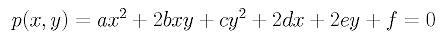If f is not zero in the above equation, we can divide the whole equation by f as shown below: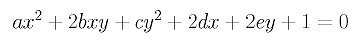Then, we actually have only five unknowns! Note that if f is zero, we already have five unknowns. Since this curve passes through P0, plugging the coordinates of P0 into the above equation yields: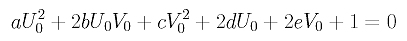The gradient of the equation is computed as follows: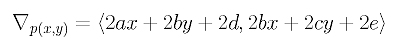The tangent line and the gradient at a P0 = (U0, V0) are perpendicular to each other. Since the gradient has a slope of (bU0+cV0+e)/ (aU0+bV0+d), the slope of the tangent line at P0 = (U0, V0) is -(aU0+bV0+d)/ (bU0+cV0+e). Since P0P1 is tangent to the conic at P0 and since the slope of line P0P1 is (V1 - V0)/(U1 - U0), these two slope values must be equal to each other. Therefore, the following must hold: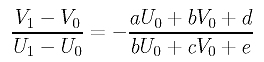Since this unknown conic also passes through P2, we have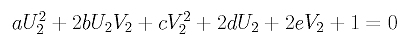Since this unknown conic is tangent to P1P2 at P2, the slope argument used earlier yields: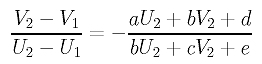Now, we have four equations, each of which is linear in the unknowns a, b, c, d and e. If we could find one more condition to generate one more needed linear equation, we will have five linear equations with five unknowns. Solving this system of linear equations yields all five coefficients and the conic curve is uniquely determined.

A very natural addition would be one more point. Plugging the coordinates of this point into the equation will give us an equation that is similar to those for control points P0 and P2. But, where is this point? This point should be inside of the triangle of the three control points so that the convex hull property can be maintained. The position of this point should also be "regular" so that we can easily change this point to produce another conic curve. One way to do this is allowing this point to be on the line segment joining P1 and the mid-point of P0P2. In this way, moving the fifth point on this line segment generates different conic curves as shown below: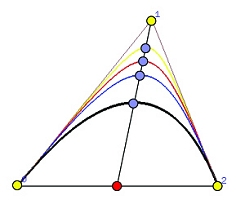### Where Is the Connection?

The connection with a NURBS curve can be seen easily. If the chosen point moves from the mid-point of P0P2 toward P1, the conic curve it helps define moves toward control point P1. On the other hand, if the point moves away from P1, the curve it helps define also moves away from P1. Therefore, this observation makes us to speculate that the position of this moving point can be controlled by the weight assigned to control P1. In fact, this is exactly the case.

Since we only need one point, which is affected by the weight of control point P1, we can use a rational Bézier curve with three control points P0, P1 and P2 with weights 1, w and 1, respectively. The weight assigned to control point P1 will control the position of the extra point. Since we use rational Bézier curve of degree 2, the coefficients are the following: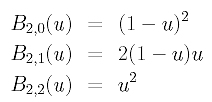The equation of this rational Bézier curve of degree 2 is simply: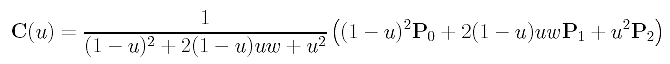To continue our discussion, for convenience, let us put P0 and P2 on the opposite sides of the x-axis with the mid-point of P0P2 being the coordinate origin. This will not affect our discussion because rational Bézier curves are projective invariant. Moreover, a simple translation followed by a rotation, both being Euclidean transformations, can get the job done. With this setting, we have P0 = -P2. Let the mid-point of P0P2 be M, and let the rational Bézier curve meets the line segment MP1 at X as shown below.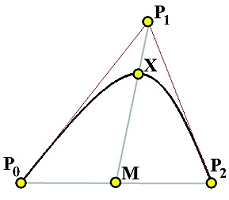Let us evaluate C(u) at u=0.5. Keep in mind that we have made P0 = -P2. A simple calculation gives us the following: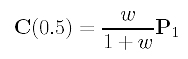Since C(0.5) and X are the same point, X and control point P1 are on the same line through the coordinate origin M, and the length of the vector X is w/(1+w) times of the length of vector P1. In other words, we have |MX|/|MP1| = w/(1+w).

We know that if w = 1, the rational Bézier curve becomes a Bézier curve, which is a parabola. In this case, since |MX|/|MP1| = w/(1+w) = 1/2, point X is the mid-point of the line segment MP1.

When is this rational Bézier curve an ellipse or a hyperbola? The answer comes from a theorem in projective geometry. From a point A outside of a given conic curve draw two tangents meeting the curve at X and Y, and an arbitrary secant line that meets the chord XY at C and the conic at B and D with B being inside of the triangle AXY as shown in the figures below. Note that if the curve is an ellipse, the order of these points is A, B, C and D; however, if it is a hyperbola, point D lies on the other branch of the curve!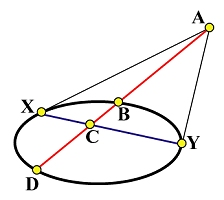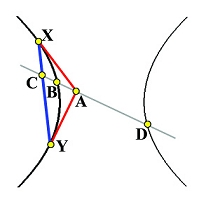(a) Ellipse (b) Hyperbola

Then, a theorem in projective geometry states that the following relation holds.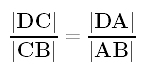Note that a correct version of this theorem should use signed distance; however, singed distance is not used here because we do not need it. A simple manipulation yields the relation below.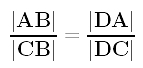Since |CA| = |CB| + |BA|, we can compute |CB|/|CA| as follows: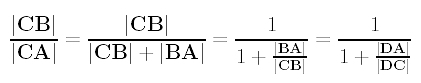If the curve is an ellipse (resp., hyperbola), we have |DA| > |DC| (resp., |DA| < |DC|), and, hence, |CB|/|CA| is less (resp., larger) than 1/2.

Return to our rational Bézier curve, we have P0=X, P1=A, P2=Y, M = C and X = C(0.5) = B. Consequently, for the case of an ellipse, we have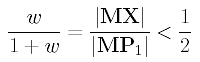This means we have an ellipse if w < 1. Similarly, we have a hyperbola if w > 1.

The following summarizes the findings:

 The rational Bézier curve defined by three non-collinear control points P0, P1 and P2 and weights 1, w and 1 is a hyperbola, a parabola or an ellipse if w is greater than, equal to, or less than 1.